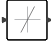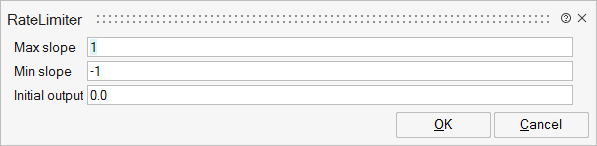# RateLimiter

This block sets a limit on the signal's derivative.Hybrid

## Description

The RateLimiter block sets a limit (maximum or minimal value) on the signal's derivative. When the derivative is larger than MaxSlope, the output is calculated by following rule

y(i) = y(i-1) + MaxSlope*step

When the derivative is smaller than MinSlope, the output is calculated by following rule

y(i) = y(i-1) + MinSlope*step

where step is the sample time in the discrete case, and integration step in the continuous case. When the derivative is between MaxSlope and MinSlope, the output is

y(i) = u(i)

When parameter InitialOutput is different from input signal when simulation starts, the initial output is calculated by following rule In case system is continuous or signal driven by non-periodic events

y(0) = u(0)

In case system is discrete, namely driven by periodic events

y(0) = y(initial) + rate*step, where rate = (u(0)-y(initial))/Ts, rate should be limited between MaxSlope and MinSlope.

This block supports double data type.

## ParametersNameLabelDescriptionData TypeValid Values

upper

Max slope

Maximum allowed derivative of input signal

Matrix

lower

Min slope

Minimum allowed derivative of input signal

Matrix

init

Initial output

Initial output of the block. Depends on the input when simulation is started.

Matrix

## Ports

NameTypeDescriptionIO TypeNumber

Port 1

explicit

input

1

Port 2

explicit

output

1

NameValueDescription

always active

no

Standard mode activated

direct-feedthrough

yes

zero-crossing

no

mode

no

continuous-time state

no

discrete-time state

no